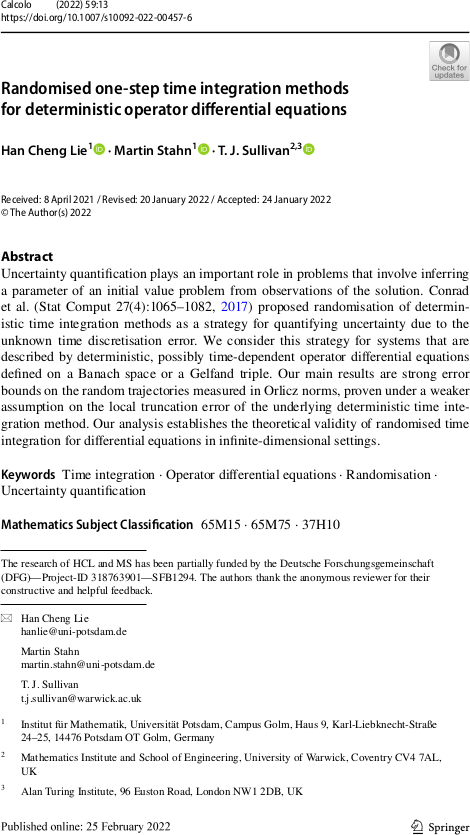# Tim Sullivan

### #stahn

Clear Search### Randomised integration for deterministic operator differential equations in Calcolo

The article “Randomised one-step time integration methods for deterministic operator differential equations” by Han Cheng Lie, Martin Stahn, and myself has just appeared in its final form in Calcolo. In this paper, we extend the analysis of Conrad et al. (2016) and Lie et al. (2019) to the case of evolutionary systems in Banach spaces or even Gel′fand triples, this being the right setting for many evolutionary partial differential equations.

H. C. Lie, M. Stahn, and T. J. Sullivan. “Randomised one-step time integration methods for deterministic operator differential equations.” Calcolo 59(1):13, 33pp., 2022. doi:10.1007/s10092-022-00457-6

Abstract. Uncertainty quantification plays an important role in applications that involve simulating ensembles of trajectories of dynamical systems. Conrad et al. (Stat. Comput., 2017) proposed randomisation of deterministic time integration methods as a strategy for quantifying uncertainty due to time discretisation. We consider this strategy for systems that are described by deterministic, possibly non-autonomous operator differential equations defined on a Banach space or a Gel′fand triple. We prove pathwise and expected error bounds on the random trajectories, given an assumption on the local truncation error of the underlying deterministic time integration and an assumption that the absolute moments of the random variables decay with the time step. Our analysis shows that the error analysis for differential equations in finite-dimensional Euclidean space carries over to infinite-dimensional settings.

Published on Friday 25 February 2022 at 17:00 UTC #publication #prob-num #lie #stahn### Randomised integration for deterministic operator differential equations

Han Cheng Lie, Martin Stahn, and I have just uploaded a preprint of our recent work “Randomised one-step time integration methods for deterministic operator differential equations” to the arXiv. In this paper, we extend the analysis of Conrad et al. (2016) and Lie et al. (2019) to the case of evolutionary systems in Banach spaces or even Gel′fand triples, this being the right setting for many evolutionary partial differential equations.

Abstract. Uncertainty quantification plays an important role in applications that involve simulating ensembles of trajectories of dynamical systems. Conrad et al. (Stat. Comput., 2017) proposed randomisation of deterministic time integration methods as a strategy for quantifying uncertainty due to time discretisation. We consider this strategy for systems that are described by deterministic, possibly non-autonomous operator differential equations defined on a Banach space or a Gel′fand triple. We prove pathwise and expected error bounds on the random trajectories, given an assumption on the local truncation error of the underlying deterministic time integration and an assumption that the absolute moments of the random variables decay with the time step. Our analysis shows that the error analysis for differential equations in finite-dimensional Euclidean space carries over to infinite-dimensional settings.

Published on Wednesday 31 March 2021 at 09:00 UTC #preprint #prob-num #lie #stahn